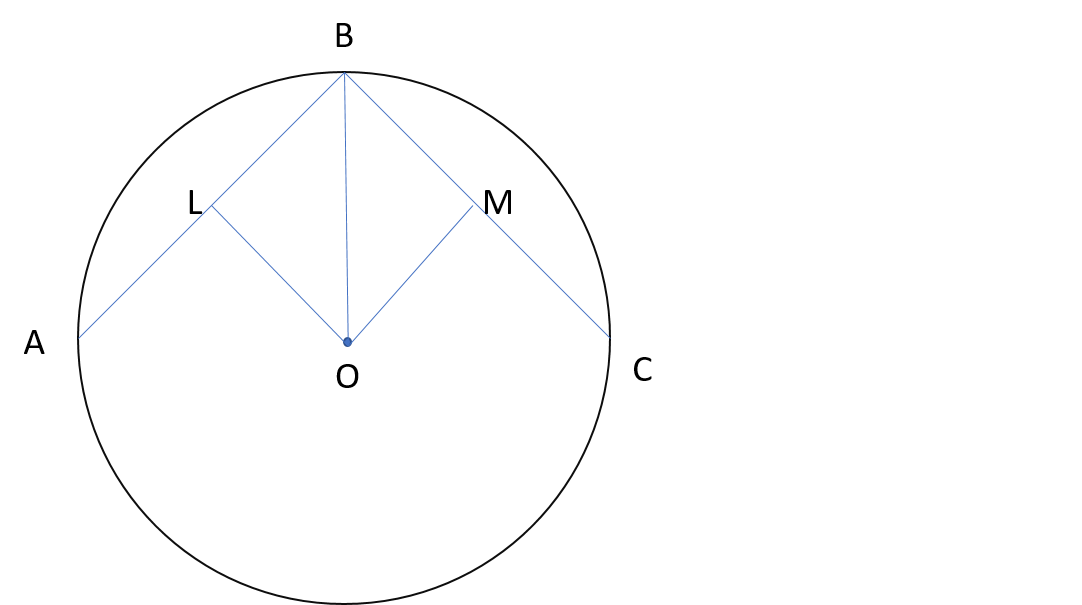# 0=1 due to a polygon?

For any $n$ sided regular polygon inscribed in a circle of radius $r$, our objective is to calculate the perimeter of the polygon.The figure represents the given situation. $O$ is the center of the circle and $AB$,$BC$ are edges of a regular polygon. $OL$ and $OM$ are perpendiculars drawn on line segments $AB$ and $BC$ respectively. Since perpendiculars from center bisect the chord, thus we have,

$OL = OM$

$OB = OB$

$\angle OLB = \angle OMB$

Therfore by RHS Criteration of congruency, $\Delta OLB \cong \Delta OMB$.

Thus by CPCT, we have

$\angle LBO = \angle OBM$

For any regular polygon of $n$ sides, each angle is given by $\frac{180(n-2)}{n}$.

Therefore, $\angle LBM = \frac{180(n-2)}{n}$. Thus $\angle LBO = \frac{180(n-2)}{2n}$.

Clearly,

$\frac{BL}{r} = cos(\frac{90(n-2)}{n}°)$

$\ BL = rcos(\frac{90(n-2)}{n}°)$ and thus,

$BC = 2BL = 2rcos(\frac{90(n-2)}{n}°)$

Since it is a regular polygon,

Perimeter = $(n)(BA) = 2nrcos(\frac{90(n-2)}{n}°)$ $.........................(1)$

But as $n$ approaches infinity the polygon tends to coincide the circle in which it is inscribed. Thus in that case the perimeter of the polygon becomes equal to the circumference of the circle in which it is inscribed.

Also As $n$ approaches infinity,

$cos(\frac{90(n-2)}{n}°)$ becomes approximately $cos(90°) = 0$ $.........................(2)$.

Also comparing the formula we obtained in equation 1 , to the circumference of circle,

$ncos(\frac{90(n-2)}{n}°) = \pi$ $.........................(3)$

From (2) and (3),

$\pi = 0$

Dividing by $\pi$ on both sides we get,

$0 =1$

Find the mistake!Note by Akshat Joshi
4 years, 5 months ago

This discussion board is a place to discuss our Daily Challenges and the math and science related to those challenges. Explanations are more than just a solution — they should explain the steps and thinking strategies that you used to obtain the solution. Comments should further the discussion of math and science.

When posting on Brilliant:

• Use the emojis to react to an explanation, whether you're congratulating a job well done , or just really confused .
• Ask specific questions about the challenge or the steps in somebody's explanation. Well-posed questions can add a lot to the discussion, but posting "I don't understand!" doesn't help anyone.
• Try to contribute something new to the discussion, whether it is an extension, generalization or other idea related to the challenge.
• Stay on topic — we're all here to learn more about math and science, not to hear about your favorite get-rich-quick scheme or current world events.

MarkdownAppears as
*italics* or _italics_ italics
**bold** or __bold__ bold
- bulleted- list
• bulleted
• list
1. numbered2. list
1. numbered
2. list
Note: you must add a full line of space before and after lists for them to show up correctly
paragraph 1paragraph 2

paragraph 1

paragraph 2

[example link](https://brilliant.org)example link
> This is a quote
This is a quote
    # I indented these lines
# 4 spaces, and now they show
# up as a code block.

print "hello world"
# I indented these lines
# 4 spaces, and now they show
# up as a code block.

print "hello world"
MathAppears as
Remember to wrap math in $$ ... $$ or $ ... $ to ensure proper formatting.
2 \times 3 $2 \times 3$
2^{34} $2^{34}$
a_{i-1} $a_{i-1}$
\frac{2}{3} $\frac{2}{3}$
\sqrt{2} $\sqrt{2}$
\sum_{i=1}^3 $\sum_{i=1}^3$
\sin \theta $\sin \theta$
\boxed{123} $\boxed{123}$

## Comments

Sort by:

Top Newest

Hint: There is something wrong with infinity

- 4 years, 5 months ago

Log in to reply

You should make this into a problem.

- 2 years, 7 months ago

Log in to reply

×

Problem Loading...

Note Loading...

Set Loading...